## Abstract

Trend estimation is a family of methods to detect and predict tendencies and trends in price series just using the history information. Moving average is a commonly used trend following trading tool. Lots of momentum trading strategies in the Forex market are based on the moving average rule, in which signals are triggered if the close is above or below the moving average. But MA has the time lag, therefore can't be used to predict the turning points of market price changes.

In this tutorial, I developed a trend following strategy which is proposed in the paper Harris R D F, Yilmaz F(2009). This strategy exploits short-term momentum in the non-linear trend component of the exchange rate which is generated by Hodrick-Prescott Filter (rather than the exchange rate itself) and uses the MA(1, 2) rule to measure this momentum. The strategy was tested on seven kinds of exchange rates and the results shows less robustness and the performance is sensitive to the change of model parameters.

## Introduction

Hodrick-Prescott Filter decomposes a time series $$y_t$$ into two components: the cyclical part (which is short-term) and the trend part (which is long term).

$y_t=x _t +c_t$

The filter is the solution to the following optimization problem for $$x_t$$

$\min _{x_t}\left[\sum_{t=1}^n(y_t-x_t)^2+\lambda\sum_{t=2}^{n-1}[(x_{t+1}-x_t)-(x_{t}-x_{t-1})^2] \right]$

The second term is the discrete derivative of the trend $$x_t$$ which characterizes the smoothness of the curve. We can rewrite the above formula in vector form:

$\min_{\bf x}{\parallel {\bf{y}}-{\bf{x}}\parallel}_2^2+\lambda {\parallel D\bf x\parallel}_2^2$

where $${\bf y}=(y_1,y_2,...,y_n),{\bf x}=(x_1,x_2,...,x_n)\in {\rm I\!R}^n$$, $$\parallel\cdot\parallel_2$$ is the Euclidean norm, and $$D$$ is the $$(n-2) \times \ n$$ matrix:

$\left[ \begin{matrix} 1 & -2 & 1 & \\ & 1 & -2 & 1 \\ & & & \ddots & & \\ & & & 1 & -2 & 1 \\ & & & & 1 & -2 & 1 \\ \end{matrix} \right]$

The solution of this optimization problem is given by solving the following linear system:

$y=(I+2\lambda D^TD)^{-1}x$

def hpfilter(self,X, lamb=1600):
X = np.asarray(X, float)
if X.ndim > 1:
X = X.squeeze()
nobs = len(X)
I = sparse.eye(nobs,nobs)
offsets = np.array([0,1,2])
data = np.repeat([[1.],[-2.],[1.]], nobs, axis=1)
K = sparse.dia_matrix((data, offsets), shape=(nobs-2,nobs))
use_umfpack = True
self.trend = spsolve(I+lamb*K.T.dot(K), X,use_umfpack=use_umfpack)
cycle = X - self.trend


## Method

This low-frequency momentum trading strategies are applied to daily data on seven kinds of exchange rates. We use five years of historical data before January 2011 for initial estimation of the trend model. Daily exchange rates for the period January 2011 to May 2017 is used for out of sample trading.

def Initialize(self):
self.SetStartDate(2011,1,1)
self.SetEndDate(2017,5,30)
self.SetCash(100000)
self.numdays = 360*5  # set the length of training period
self.n,self.m = 2, 1
self.trend = None
self.SetBenchmark(self.syl)
self.MA_rules = None
history = self.History(self.numdays,Resolution.Daily)
self.close = [slice[self.syl].Close for slice in history]


#### Step 1: Calibrating the Filter Smoothing Parameter λ

The Ravn–Uhlig rule is commonly used to set the smoothing parameter $$\lambda$$ in HP filter and must be greater than 0. It is adjusted by the changing frequency of observations and must be greater than 0. Hodrick and Prescott (1997) recommended setting $$\lambda$$ to 1,600 for quarterly data. The Ravn–Uhlig rule sets  $$\lambda = 1600p^4$$ , where p is the number of periods per quarter. As for our daily exchange rate data, we should have set $$\lambda$$ to be $$1600\times (30 \times 4)^4$$. But when we use this value as the $$\lambda$$, the curve is almost a straight line since the trend becomes smoother as $$\lambda \rightarrow \infty$$. In order to avoid excessive smoothing, we gradually decrease the $$\lambda$$ and draw the smoothing curve. Below is the chart of EURUSD daily price from the year 2010 to 2011. t100 denotes the trend component after filter with the parameter $$\lambda=100$$.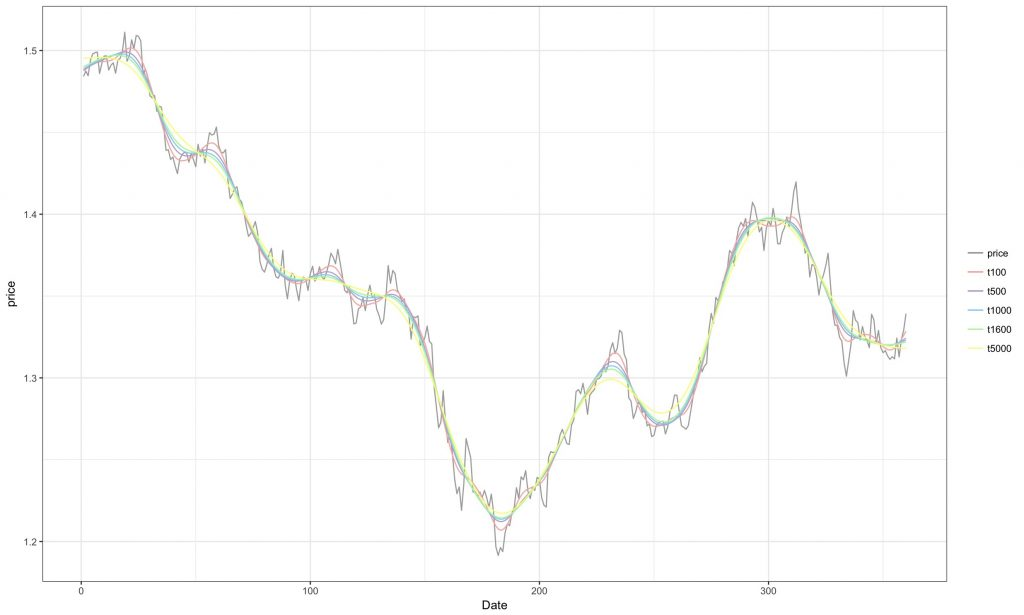If we just plot the curve for the first 100 days, we find that the smaller the $$\lambda$$, the more apparent the trend. The curve does not change too much as the $$\lambda$$ smaller than 100. Thus here we choose  $$\lambda=100$$ to extract the trend of daily price data. This trend is our low-frequency component.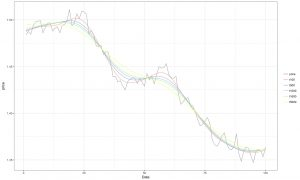Out-of-sample EUR/USD Trend Estimation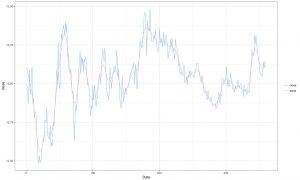#### Step 2: Setting up the Moving Average Rule

Moving average (MA) rules are very commonly used to generate buy and sell signals from data on the spot exchange rate. The MA rule compares a short-run moving average of the current and lagged exchange rate with a long-run moving average.

$MA(m,n)=\frac{1}{m}\sum_{i=0}^{m-1}S_{t-i}-\frac{1}{n}\sum_{i=0}^{n-1}S_{t-i}$

For HP filter, the non-linear trend is estimated recursively as the paper did. The initial estimation was undertaken using 3 years history data before 2011. The estimation period is then rolled forward each day through the trading period from January 2011 to May 2017.

#### Step 3: Generating the Trading Signals

We generate buy and sell signals by applying an $$MA(1, 2)$$ rule to the estimated low-frequency component. For $$MA(m,n)$$, $$m$$ must be 1 which denotes the current value of low-frequency component. $$n$$ should be small since large $$n$$ would generate large time lag, the judgment of turning points is not accurate.

A buy signal is generated when the current day’s low-frequency trend is higher than the last day’s low-frequency trend and a sell signal is generated when it is lower.

def OnData(self,data):
self.close.append(self.Portfolio[self.syl].Price)
self.hpfilter(self.close[-self.numdays:len(self.close)+1], 100)
self.MA_rules_today = (np.mean(self.trend[-self.m : len(self.trend)]) - np.mean(self.trend[-self.n : len(self.trend)]))
self.MA_rules_yesterday = (np.mean(self.trend[-self.m-1: len(self.trend)-1]) - np.mean(self.trend[-self.n-1 : len(self.trend)-1]))
holdings = self.Portfolio[self.syl].Quantity

if self.MA_rules_today > 0 and self.MA_rules_yesterday < 0:
self.SetHoldings(self.syl, 1)
elif self.MA_rules_today < 0 and self.MA_rules_yesterday > 0:
self.SetHoldings(self.syl, -1)


Trading Signals when $$\lambda=1600$$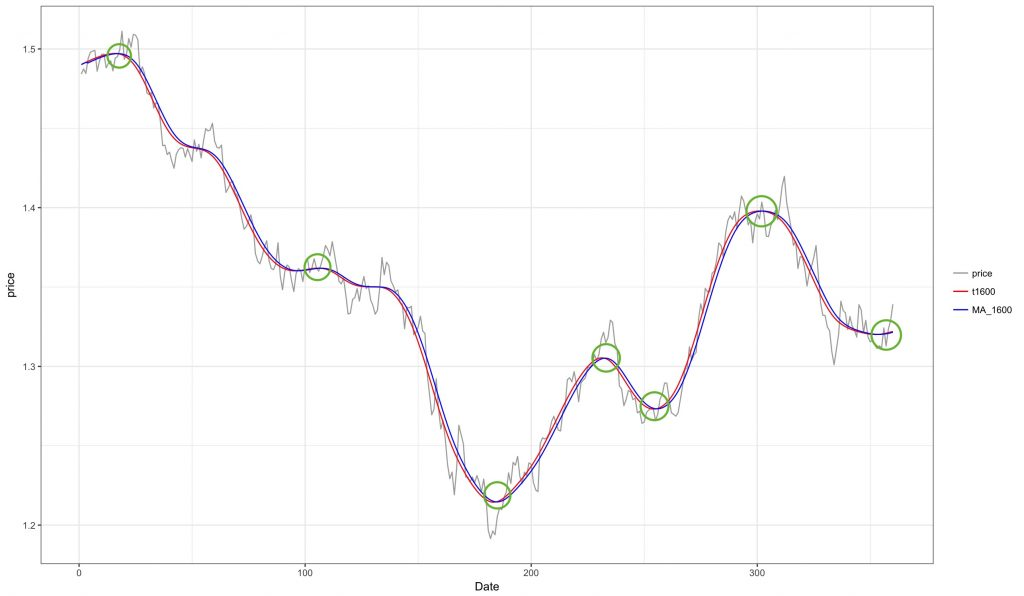Trading Signals when $$\lambda=100$$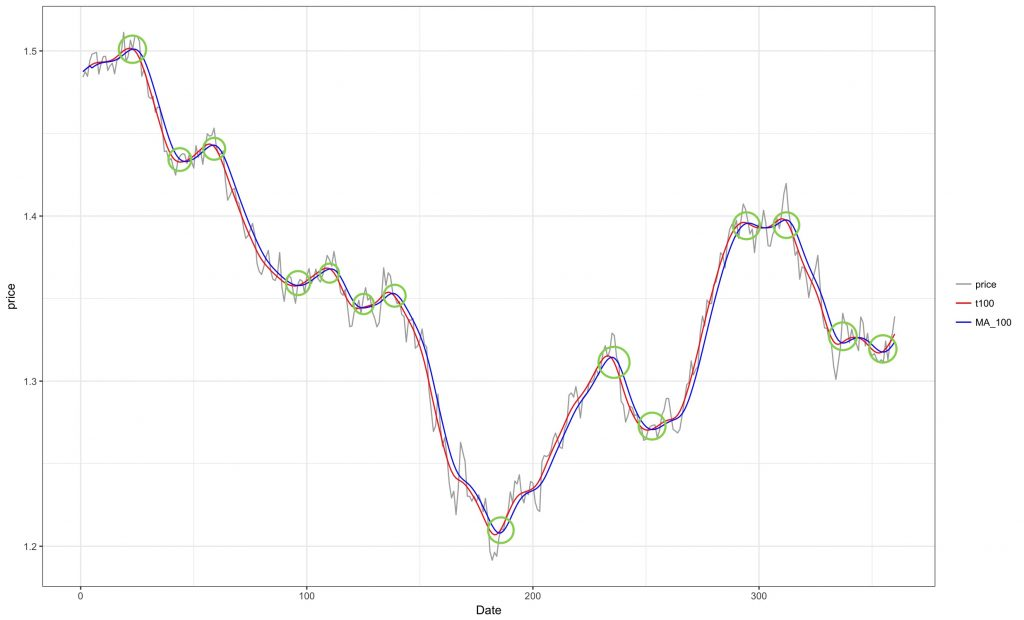The above charts are the in-sample trading signals after applying $$MA$$ rules on the low-frequency component. The trend curve is more smooth with larger $$\lambda$$. Thus when we applied $$MA$$ rules, a less smooth trend will trigger more trading opportunities.

## Summary

The following table shows the strategy performance for different Forex pairs from 1/1/2011 to 5/30/2017:

Pair Sharpe Ratio Total Trades Annual Return Max Drawdown
EURUSD -0.309 14 -3.652% 23.700%
USDCHF 0.044 14 -0.154% 28.500%
EURGBP 0.480 15 4.035% 21.800%
USDNOK 0.210 13 2.187% 19.700%
USDZAR 0.166 10 1.042% 52.900%

The table reports the strategy performance statistics during six and a half years backtesting period. From the table we can see, most of them have the higher maximum drawdown. The number of total trades is small because we applied $$MA$$ rules on the smoothed trend component.  As the author indicated in the paper, we still find that the performance of this strategy is very sensitive to the choice of lag parameters in $$MA$$ rules and in a non-monotonic way.

The strategy does not generate more stable profits in Forex market generally. That might because that the HP filter technique was designed to be viewed as a trend curve through the entire set of data. When we applied it in trading strategy, the entry of new data into the filter model can cause the trend line to change the trend through past data, makes it harder to identify the trend accurately.

## Reference

1. Harris R D F, Yilmaz F. A momentum trading strategy based on the low-frequency component of the exchange rate[J]. Journal of Banking & Finance, 2009, 33(9): 1575-1585. online copy
2. Dao T L. Momentum Strategies with L1 Filter[J]. Browser Download This Paper, 2014. online copy